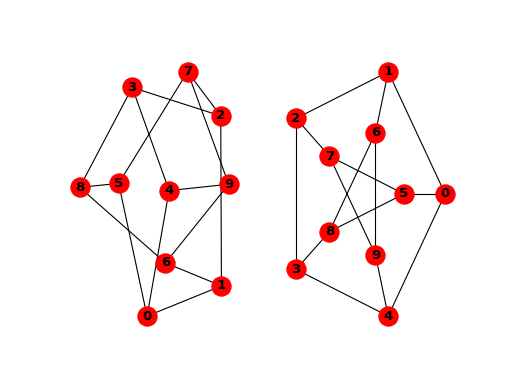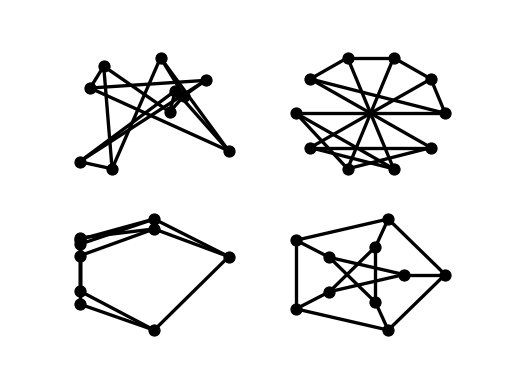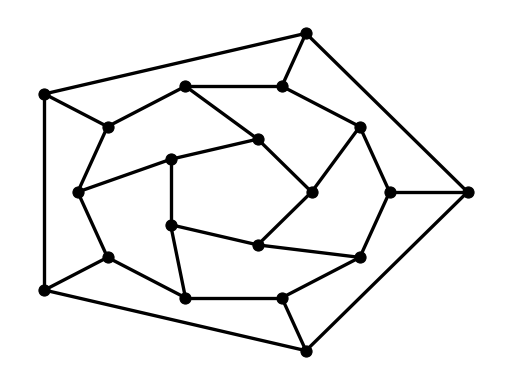Warning

This documents an unmaintained version of NetworkX. Please upgrade to a maintained version and see the current NetworkX documentation.

# Tutorial¶

## Creating a graph¶

Create an empty graph with no nodes and no edges.

>>> import networkx as nx
>>> G = nx.Graph()


By definition, a Graph is a collection of nodes (vertices) along with identified pairs of nodes (called edges, links, etc). In NetworkX, nodes can be any hashable object e.g., a text string, an image, an XML object, another Graph, a customized node object, etc.

Note

Python’s None object should not be used as a node as it determines whether optional function arguments have been assigned in many functions.

## Nodes¶

The graph G can be grown in several ways. NetworkX includes many graph generator functions and facilities to read and write graphs in many formats. To get started though we’ll look at simple manipulations. You can add one node at a time,

>>> G.add_node(1)


>>> G.add_nodes_from([2, 3])


or add any iterable container of nodes. You can also add nodes along with node attributes if your container yields 2-tuples (node, node_attribute_dict). Node attributes are discussed furether below.

>>> H = nx.path_graph(10)


Note that G now contains the nodes of H as nodes of G. In contrast, you could use the graph H as a node in G.

>>> G.add_node(H)


The graph G now contains H as a node. This flexibility is very powerful as it allows graphs of graphs, graphs of files, graphs of functions and much more. It is worth thinking about how to structure your application so that the nodes are useful entities. Of course you can always use a unique identifier in G and have a separate dictionary keyed by identifier to the node information if you prefer.

Note

You should not change the node object if the hash depends on its contents.

## Edges¶

G can also be grown by adding one edge at a time,

>>> G.add_edge(1, 2)
>>> e = (2, 3)
>>> G.add_edge(*e)  # unpack edge tuple*


by adding a list of edges,

>>> G.add_edges_from([(1, 2), (1, 3)])


or by adding any ebunch of edges. An ebunch is any iterable container of edge-tuples. An edge-tuple can be a 2-tuple of nodes or a 3-tuple with 2 nodes followed by an edge attribute dictionary, e.g., (2, 3, {'weight': 3.1415}). Edge attributes are discussed further below

>>> G.add_edges_from(H.edges)


There are no complaints when adding existing nodes or edges. For example, after removing all nodes and edges,

>>> G.clear()


we add new nodes/edges and NetworkX quietly ignores any that are already present.

>>> G.add_edges_from([(1, 2), (1, 3)])


At this stage the graph G consists of 8 nodes and 2 edges, as can be seen by:

>>> G.number_of_nodes()
8
>>> G.number_of_edges()
3


We can examine the nodes and edges. Four basic graph properties facilitate reporting: G.nodes, G.edges, G.adj and G.degree. These are set-like views of the nodes, edges, neighbors (adjacencies), and degrees of nodes in a graph. They offer a continually updated read-only view into the graph structure. They are also dict-like in that you can look up node and edge data attributes via the views and iterate with data attributes using methods .items(), .data('span'). If you want a specific container type instead of a view, you can specify one. Here we use lists, though sets, dicts, tuples and other containers may be better in other contexts.

>>> list(G.nodes)
['a', 1, 2, 3, 'spam', 'm', 'p', 's']
>>> list(G.edges)
[(1, 2), (1, 3), (3, 'm')]
[2, 3]
>>> G.degree  # the number of edges incident to 1
2


One can specify to report the edges and degree from a subset of all nodes using an nbunch. An nbunch is any of: None (meaning all nodes), a node, or an iterable container of nodes that is not itself a node in the graph.

>>> G.edges([2, 'm'])
EdgeDataView([(2, 1), ('m', 3)])
>>> G.degree([2, 3])
DegreeView({2: 1, 3: 2})


One can remove nodes and edges from the graph in a similar fashion to adding. Use methods Graph.remove_node(), Graph.remove_nodes_from(), Graph.remove_edge() and Graph.remove_edges_from(), e.g.

>>> G.remove_node(2)
>>> G.remove_nodes_from("spam")
>>> list(G.nodes)
[1, 3, 'spam']
>>> G.remove_edge(1, 3)


When creating a graph structure by instantiating one of the graph classes you can specify data in several formats.

>>> G.add_edge(1, 2)
>>> H = nx.DiGraph(G)   # create a DiGraph using the connections from G
>>> list(H.edges())
[(1, 2), (2, 1)]
>>> edgelist = [(0, 1), (1, 2), (2, 3)]
>>> H = nx.Graph(edgelist)


## What to use as nodes and edges¶

You might notice that nodes and edges are not specified as NetworkX objects. This leaves you free to use meaningful items as nodes and edges. The most common choices are numbers or strings, but a node can be any hashable object (except None), and an edge can be associated with any object x using G.add_edge(n1, n2, object=x).

As an example, n1 and n2 could be protein objects from the RCSB Protein Data Bank, and x could refer to an XML record of publications detailing experimental observations of their interaction.

We have found this power quite useful, but its abuse can lead to unexpected surprises unless one is familiar with Python. If in doubt, consider using convert_node_labels_to_integers() to obtain a more traditional graph with integer labels.

## Accessing edges and neighbors¶

In addition to the views Graph.edges(), and Graph.adj(), access to edges and neighbors is possible using subscript notation.

>>> G  # same as G.adj
AtlasView({2: {}})
>>> G
{}
>>> G.edges[1, 2]
{}


You can get/set the attributes of an edge using subscript notation if the edge already exists.

>>> G.add_edge(1, 3)
>>> G['color'] = "blue"
>>> G.edges[1, 2]['color'] = "red"


Fast examination of all (node, adjacency) pairs is achieved using G.adjacency(), or G.adj.items(). Note that for undirected graphs, adjacency iteration sees each edge twice.

>>> FG = nx.Graph()
>>> FG.add_weighted_edges_from([(1, 2, 0.125), (1, 3, 0.75), (2, 4, 1.2), (3, 4, 0.375)])
>>> for n, nbrs in FG.adj.items():
...    for nbr, eattr in nbrs.items():
...        wt = eattr['weight']
...        if wt < 0.5: print('(%d, %d, %.3f)' % (n, nbr, wt))
(1, 2, 0.125)
(2, 1, 0.125)
(3, 4, 0.375)
(4, 3, 0.375)


>>> for (u, v, wt) in FG.edges.data('weight'):
...     if wt < 0.5: print('(%d, %d, %.3f)' % (u, v, wt))
(1, 2, 0.125)
(3, 4, 0.375)


## Adding attributes to graphs, nodes, and edges¶

Attributes such as weights, labels, colors, or whatever Python object you like, can be attached to graphs, nodes, or edges.

Each graph, node, and edge can hold key/value attribute pairs in an associated attribute dictionary (the keys must be hashable). By default these are empty, but attributes can be added or changed using add_edge, add_node or direct manipulation of the attribute dictionaries named G.graph, G.nodes, and G.edges for a graph G.

### Graph attributes¶

Assign graph attributes when creating a new graph

>>> G = nx.Graph(day="Friday")
>>> G.graph
{'day': 'Friday'}


Or you can modify attributes later

>>> G.graph['day'] = "Monday"
>>> G.graph
{'day': 'Monday'}


### Node attributes¶

Add node attributes using add_node(), add_nodes_from(), or G.nodes

>>> G.add_node(1, time='5pm')
>>> G.nodes
{'time': '5pm'}
>>> G.nodes['room'] = 714
>>> G.nodes.data()
NodeDataView({1: {'room': 714, 'time': '5pm'}, 3: {'time': '2pm'}})


Note that adding a node to G.nodes does not add it to the graph, use G.add_node() to add new nodes. Similarly for edges.

### Edge Attributes¶

Add/change edge attributes using add_edge(), add_edges_from(), or subscript notation.

>>> G.add_edge(1, 2, weight=4.7 )
>>> G.add_edges_from([(3, 4), (4, 5)], color='red')
>>> G.add_edges_from([(1, 2, {'color': 'blue'}), (2, 3, {'weight': 8})])
>>> G['weight'] = 4.7
>>> G.edges[3, 4]['weight'] = 4.2


The special attribute weight should be numeric as it is used by algorithms requiring weighted edges.

## Directed graphs¶

The DiGraph class provides additional properties specific to directed edges, e.g., DiGraph.out_edges(), DiGraph.in_degree(), DiGraph.predecessors(), DiGraph.successors() etc. To allow algorithms to work with both classes easily, the directed versions of neighbors() is equivalent to successors() while degree reports the sum of in_degree and out_degree even though that may feel inconsistent at times.

>>> DG = nx.DiGraph()
>>> DG.add_weighted_edges_from([(1, 2, 0.5), (3, 1, 0.75)])
>>> DG.out_degree(1, weight='weight')
0.5
>>> DG.degree(1, weight='weight')
1.25
>>> list(DG.successors(1))

>>> list(DG.neighbors(1))



Some algorithms work only for directed graphs and others are not well defined for directed graphs. Indeed the tendency to lump directed and undirected graphs together is dangerous. If you want to treat a directed graph as undirected for some measurement you should probably convert it using Graph.to_undirected() or with

>>> H = nx.Graph(G)  # convert G to undirected graph


## Multigraphs¶

NetworkX provides classes for graphs which allow multiple edges between any pair of nodes. The MultiGraph and MultiDiGraph classes allow you to add the same edge twice, possibly with different edge data. This can be powerful for some applications, but many algorithms are not well defined on such graphs. Where results are well defined, e.g., MultiGraph.degree() we provide the function. Otherwise you should convert to a standard graph in a way that makes the measurement well defined.

>>> MG = nx.MultiGraph()
>>> MG.add_weighted_edges_from([(1, 2, 0.5), (1, 2, 0.75), (2, 3, 0.5)])
>>> dict(MG.degree(weight='weight'))
{1: 1.25, 2: 1.75, 3: 0.5}
>>> GG = nx.Graph()
>>> for n, nbrs in MG.adjacency():
...    for nbr, edict in nbrs.items():
...        minvalue = min([d['weight'] for d in edict.values()])
...        GG.add_edge(n, nbr, weight = minvalue)
...
>>> nx.shortest_path(GG, 1, 3)
[1, 2, 3]


## Graph generators and graph operations¶

In addition to constructing graphs node-by-node or edge-by-edge, they can also be generated by

1. Applying classic graph operations, such as:

subgraph(G, nbunch)      - induced subgraph view of G on nodes in nbunch
union(G1,G2)             - graph union
disjoint_union(G1,G2)    - graph union assuming all nodes are different
cartesian_product(G1,G2) - return Cartesian product graph
compose(G1,G2)           - combine graphs identifying nodes common to both
complement(G)            - graph complement
create_empty_copy(G)     - return an empty copy of the same graph class
convert_to_undirected(G) - return an undirected representation of G
convert_to_directed(G)   - return a directed representation of G

2. Using a call to one of the classic small graphs, e.g.,

>>> petersen = nx.petersen_graph()
>>> tutte = nx.tutte_graph()
>>> maze = nx.sedgewick_maze_graph()
>>> tet = nx.tetrahedral_graph()

1. Using a (constructive) generator for a classic graph, e.g.,
>>> K_5 = nx.complete_graph(5)
>>> K_3_5 = nx.complete_bipartite_graph(3, 5)
>>> barbell = nx.barbell_graph(10, 10)
>>> lollipop = nx.lollipop_graph(10, 20)

1. Using a stochastic graph generator, e.g.,
>>> er = nx.erdos_renyi_graph(100, 0.15)
>>> ws = nx.watts_strogatz_graph(30, 3, 0.1)
>>> ba = nx.barabasi_albert_graph(100, 5)
>>> red = nx.random_lobster(100, 0.9, 0.9)

1. Reading a graph stored in a file using common graph formats, such as edge lists, adjacency lists, GML, GraphML, pickle, LEDA and others.
>>> nx.write_gml(red, "path.to.file")


For details on graph formats see Reading and writing graphs and for graph generator functions see Graph generators

## Analyzing graphs¶

The structure of G can be analyzed using various graph-theoretic functions such as:

>>> G = nx.Graph()
>>> list(nx.connected_components(G))
[set([1, 2, 3]), set(['spam'])]
>>> sorted(d for n, d in G.degree())
[0, 1, 1, 2]
>>> nx.clustering(G)
{1: 0, 2: 0, 3: 0, 'spam': 0}


Some functions with large output iterate over (node, value) 2-tuples. These are easily stored in a dict structure if you desire.

>>> sp = dict(nx.all_pairs_shortest_path(G))
>>> sp
{1: [3, 1], 2: [3, 1, 2], 3: }


See Algorithms for details on graph algorithms supported.

## Drawing graphs¶

NetworkX is not primarily a graph drawing package but basic drawing with Matplotlib as well as an interface to use the open source Graphviz software package are included. These are part of the networkx.drawing module and will be imported if possible.

First import Matplotlib’s plot interface (pylab works too)

>>> import matplotlib.pyplot as plt


You may find it useful to interactively test code using ipython -pylab, which combines the power of ipython and matplotlib and provides a convenient interactive mode.

To test if the import of networkx.drawing was successful draw G using one of

>>> G = nx.petersen_graph()
>>> plt.subplot(121)
<matplotlib.axes._subplots.AxesSubplot object at ...>
>>> nx.draw(G, with_labels=True, font_weight='bold')
>>> plt.subplot(122)
<matplotlib.axes._subplots.AxesSubplot object at ...>
>>> nx.draw_shell(G, nlist=[range(5, 10), range(5)], with_labels=True, font_weight='bold')


(png, hires.png, pdf)when drawing to an interactive display. Note that you may need to issue a Matplotlib

>>> plt.show()


command if you are not using matplotlib in interactive mode (see Matplotlib FAQ ).

>>> options = {
...     'node_color': 'black',
...     'node_size': 100,
...     'width': 3,
... }
>>> plt.subplot(221)
<matplotlib.axes._subplots.AxesSubplot object at ...>
>>> nx.draw_random(G, **options)
>>> plt.subplot(222)
<matplotlib.axes._subplots.AxesSubplot object at ...>
>>> nx.draw_circular(G, **options)
>>> plt.subplot(223)
<matplotlib.axes._subplots.AxesSubplot object at ...>
>>> nx.draw_spectral(G, **options)
>>> plt.subplot(224)
<matplotlib.axes._subplots.AxesSubplot object at ...>
>>> nx.draw_shell(G, nlist=[range(5,10), range(5)], **options)


(png, hires.png, pdf)You can find additional options via draw_networkx() and layouts via layout. You can use multiple shells with draw_shell().

>>> G = nx.dodecahedral_graph()
>>> shells = [[2, 3, 4, 5, 6], [8, 1, 0, 19, 18, 17, 16, 15, 14, 7], [9, 10, 11, 12, 13]]
>>> nx.draw_shell(G, nlist=shells, **options)


(png, hires.png, pdf)To save drawings to a file, use, for example

>>> nx.draw(G)
>>> plt.savefig("path.png")


writes to the file path.png in the local directory. If Graphviz and PyGraphviz or pydot, are available on your system, you can also use nx_agraph.graphviz_layout(G) or nx_pydot.graphviz_layout(G) to get the node positions, or write the graph in dot format for further processing.

>>> from networkx.drawing.nx_pydot import write_dot
>>> pos = nx.nx_agraph.graphviz_layout(G)
>>> nx.draw(G, pos=pos)
>>> write_dot(G, 'file.dot')